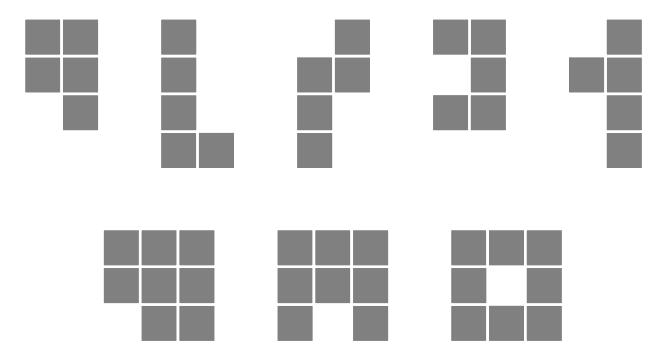# 2916. Lattice Animals

Lattice animal is a set of connected sites on a lattice. Lattice animals on a square lattice are especially popular subject of study and are also known as polyominoes. Polyomino is usually represented as a set of sidewise connected squares. Polyomino with $n$ squares is called $n$-polyomino.

In this problem you are to find a number of distinct free $n$-polyominoes that fit into rectangle $w \times h$. Free polyominoes can be rotated and flipped over, so that their rotations and mirror images are considered to be the same.

For example, there are 5 different pentominoes (5-polyominoes) that fit into $2 \times 4$ rectangle and 3 different octominoes (8-polyominoes) that fit into $3 \times 3$ rectangle.### 输入格式

The input file consists of a single line with 3 integer numbers $n$, $w$, and $h$ ($1 \le n \le 10$, $1 \le w, h \le n$).

### 输出格式

Write to the output file a single integer number — the number of distinct free $n$-polyominoes that fit into rectangle $w \times h$.

### 样例

Input
5 1 4

Output
0

Input
5 2 4

Output
5

Input
5 3 4

Output
11

Input
5 5 5

Output
12

Input
8 3 3

Output
3


4 人解决，25 人已尝试。

4 份提交通过，共有 53 份提交。

9.2 EMB 奖励。